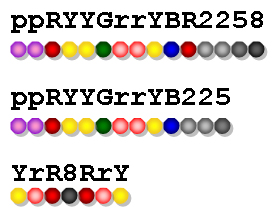2021/8/21 20:02:04 浏览：

For the sake of simplicity, let’s use the characters in the ranges [0-9], [a-z], and [A-Z] to represent the colors. For example, the 3rd string in Figure 1 is the one that Eva would like to make. Then the 1st string is okay since it contains all the necessary beads with 8 extra ones; yet the 2nd one is not since there is no black bead and one less red bead.Figure 1

Input Specification

Each input file contains one test case. Each case gives in two lines the strings of no more than 1000 beads which belong to the shop owner and Eva, respectively.

Output Specification

For each test case, print your answer in one line. If the answer is Yes, then also output the number of extra beads Eva has to buy; or if the answer is No, then also output the number of beads missing from the string. There must be exactly 1 space between the answer and the number.

ppRYYGrrYBR2258
YrR8RrY

Yes 8

ppRYYGrrYB225
YrR8RrY

No 2

题意

Eva买线，但老板只整盒整盒的卖，要求计算Eva买固定颜色的线盒需要多买的颜色数或者缺的颜色数。

代码

#include <iostream>
using namespace std;
int c1= {0},c2= {0};
int main() {
string s,n;	//s已有的、n需要的
int extra=0,missing=0;
cin >> s >> n;
for(int i=0; i<s.length(); i++) {
c1[s[i]]++;
}
for(int i=0; i<n.length(); i++) {
c2[n[i]]++;
}
for(int i=0; i<256; i++) {
if(c1[i]>c2[i]) {
extra += c1[i]-c2[i];
} else if(c1[i]<c2[i]) {
missing += c2[i] -c1[i];
}
}
if(missing > 0) {
printf("No %d",missing);
} else {
printf("Yes %d",extra);
}
return 0;
}